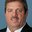Question-and-Answer Resource for the Building Energy Modeling Community
Get started with the Help page

# How is latent cooling energy/rate calculated in ideal-loads-air-system with humidistat ?I am modelling the IdealLoadsAirSystem in energyplus with humidistat set to 50%. What does the output report Zone Ideal Loads Supply Air Latent Cooling Rate [W] actually mean? When the indoor RH is less than 50% (say 47%), I would have expected this value to be zero. The only time when this value is zero is when the sensible rate is zero and the indoor RH is less than setpoint. Does this output also include whatever latent cooling happens due to the supply air? If yes, how is it calculated - I did not notice a constant SHR. Thanks, -Rohini

edit retag close merge delete

Sort by » oldest newest most voted

In general the latent cooling rate is getting calculated as Richard mentioned. Here are the details of how the latent load is getting calculated, when you have a humidistat. Case 1 - Non-OA system, no dehumidification load: In this case the Zone Predicted Moisture Load to Dehumidifying Setpoint Moisture Transfer Rate [kgWater/s] is a positive value. The supply air humidity ratio is whatever is specified in the ideal loads component. Return values are same as the zone values. The latent cooling rate is calculated from this. Case 2 - Non-OA system, yes dehumidification load: In this case the Zone Predicted Moisture Load to Dehumidifying Setpoint Moisture Transfer Rate [kgWater/s] is a negative value - i.e. there is a latent load on the system. The supply air humidity ratio is set to a value that will satisfy this latent load. Return values are same as the zone values. The latent cooling rate is calculated from this. In this case the Supply Air Latent Cooling Rate is same as the predicted moisture load (Moisture transfer rate x latent heat of vaporization). Case 3 - When OA is used: In this case the supply air humidity is decided as above. The latent cooling rate calculations are based on these supply conditions and the mixed air conditions. So the cooling rates are different from the predicted load (unless the OA is the same exact condition as return air).

moreThe report variable representing the latent cooling energy or rate describes system performance with regard to moisture energy transfer. There are multiple reports for the ideal loads air system. The cooling components of latent heat transfer are shown below.

Zone Ideal Loads Supply Air Latent Cooling Rate [W]
Zone Ideal Loads Zone Latent Cooling Rate [W]
Zone Ideal Loads Outdoor Air Latent Cooling Rate [W]


The calculations for latent performance are similar for each of these reports. The total enthalpic energy transfer rate is calculated and then the sensible (temperature) component is subtracted from this value. This value is reported regardless of humidistat usage.

$$Q_{lat} = [ \dot{m} \times ( H_{supply} - H_{mixedair} ) ] - Q_{sens}$$

where:
$\dot{m}$ is the zone air mass flow rate,
$H_{supply}$ is the ideal loads air system output directed to the zone,
$H_{mixedair}$ is the air enthalpy entering the ideal loads air system (including outdoor air) and
$Q_{sens}=\dot{m}\times c_p \times (T_{zoneinlet}-T_{zone})$.

more